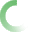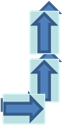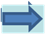﻿ HydroPower_web.xlsxPROGRAM: HYDROPOWER

>DATA<
Flow= CU FT / SEC   K FACTOR TRIAL VALUES
Wt. of H2O= LB/CU FT     K= ft hp
Turbine effiency=     Kaplan 1250
Generator effiency=Propeller 1150
K factor = TRIAL VALUE Francis 1050
>RESULTS<Turbine Power= HP
Generator Power= KW
Ns Specfic speed= FROM K FACTOR
Rotational speed= RPM
Number of poles= MUST BE INTERGER DIVISIBLE BY 2 Adjust K factor
Velocity ratio= FRANCIS
Velocity ratio = KAPLAN OR PROP
Runner Diameter= INCHES - FRANCIS
Runner Diameter= INCHES - KAPLAN OR PROP
To use: Enter Data, inputing head and flow to reach desired power output, then use trial K factor for type of turbine desired, then adjust K factor until number of poles is divisble by 2 (preferably by 4) to yield a synchronous speed at 60 HZ, while specific speed and head falls within table values given below. Generally, a slower ROTATIONAL speed is desired.

Specific Speed Range Head Range (ft)   No. of Poles Sync. Speed at 60 HZ
Kaplan 70 to 225 10 to 130
Propeller 70 to 225 10 to 250
Francis 15 to 85 50 to 850